## Overview

In addition to Model based Imputation Methods (see vignette("modelImp")) the VIM package also presents donor based imputation methods, namely Hot-Deck Imputation, k-Nearest Neighbour Imputation and fast matching/imputation based on categorical variable.

This vignette showcases the functions hotdeck() and kNN(), which can both be used to generate imputations for several variables in a dataset. Moreover, the function matchImpute() is presented, which is in contrast a imputation method based on categorical variables.

## Data

The following example demonstrates the functionality of hodeck() and kNN() using a subset of sleep. The columns have been selected deliberately to include some interactions between the missing values.

library(VIM)
dataset <- sleep[, c("Dream", "NonD", "BodyWgt", "Span")]
dataset$BodyWgt <- log(dataset$BodyWgt)
dataset$Span <- log(dataset$Span)
aggr(dataset)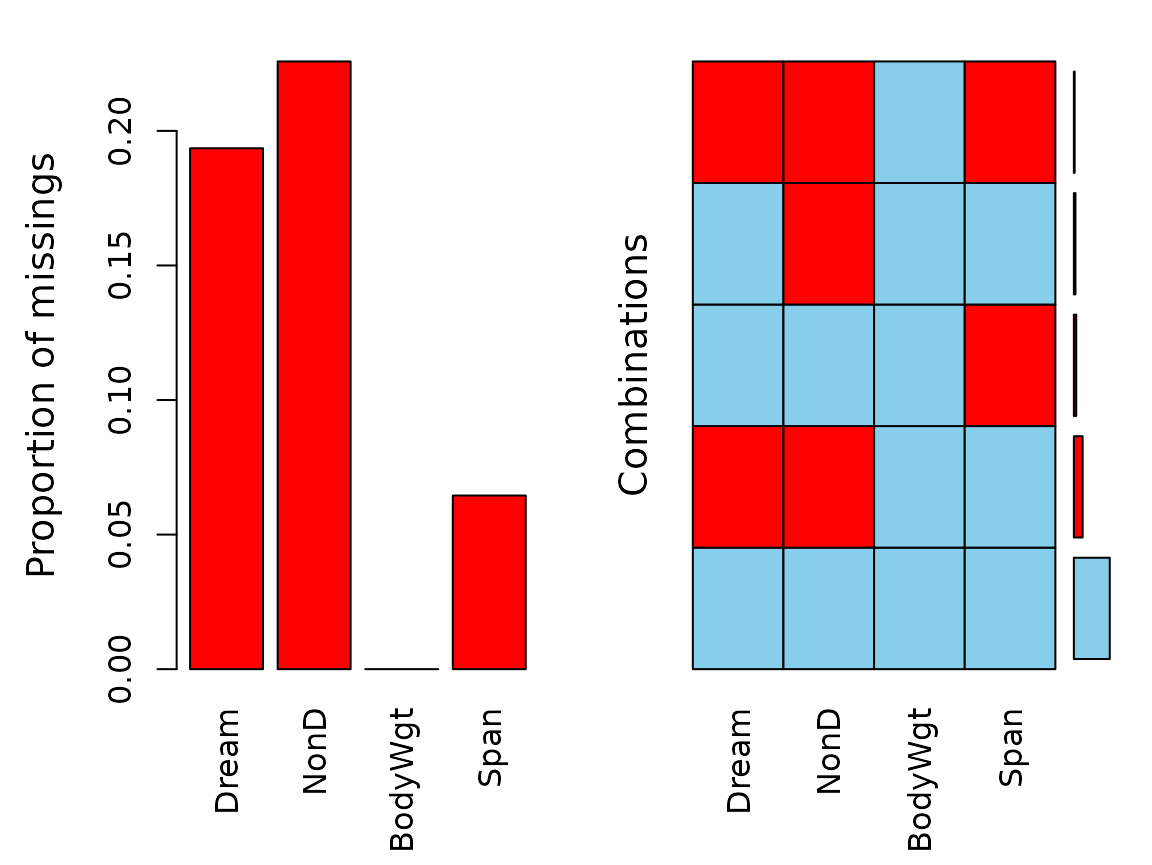The plot indicates several missing values in Dream, NonD, and Span.

sapply(dataset, function(x)sum(is.na(x)))
#>   Dream    NonD BodyWgt    Span
#>      12      14       0       4

## Imputation

The call of the functions is straightforward. We will start by just imputing NonD based on the other variables. Besides imputing missing variables for a single variable, these functions also support imputation of multiple variables. For matchImpute() suitable donors are searched based on matching of the categorical variables.

imp_hotdeck <- hotdeck(dataset, variable = "NonD")  # hotdeck imputation
imp_knn <- kNN(dataset, variable = "NonD") # kNN imputation
#>      Dream    BodyWgt       Span      Dream    BodyWgt       Span
#>  0.0000000 -5.2983174  0.6931472  6.6000000  8.8029735  4.6051702
imp_match <- matchImpute(dataset, variable = "NonD", match_var = c("BodyWgt","Span")) # match imputation
aggr(imp_knn, delimiter = "_imp")
aggr(imp_match, delimiter = "_imp")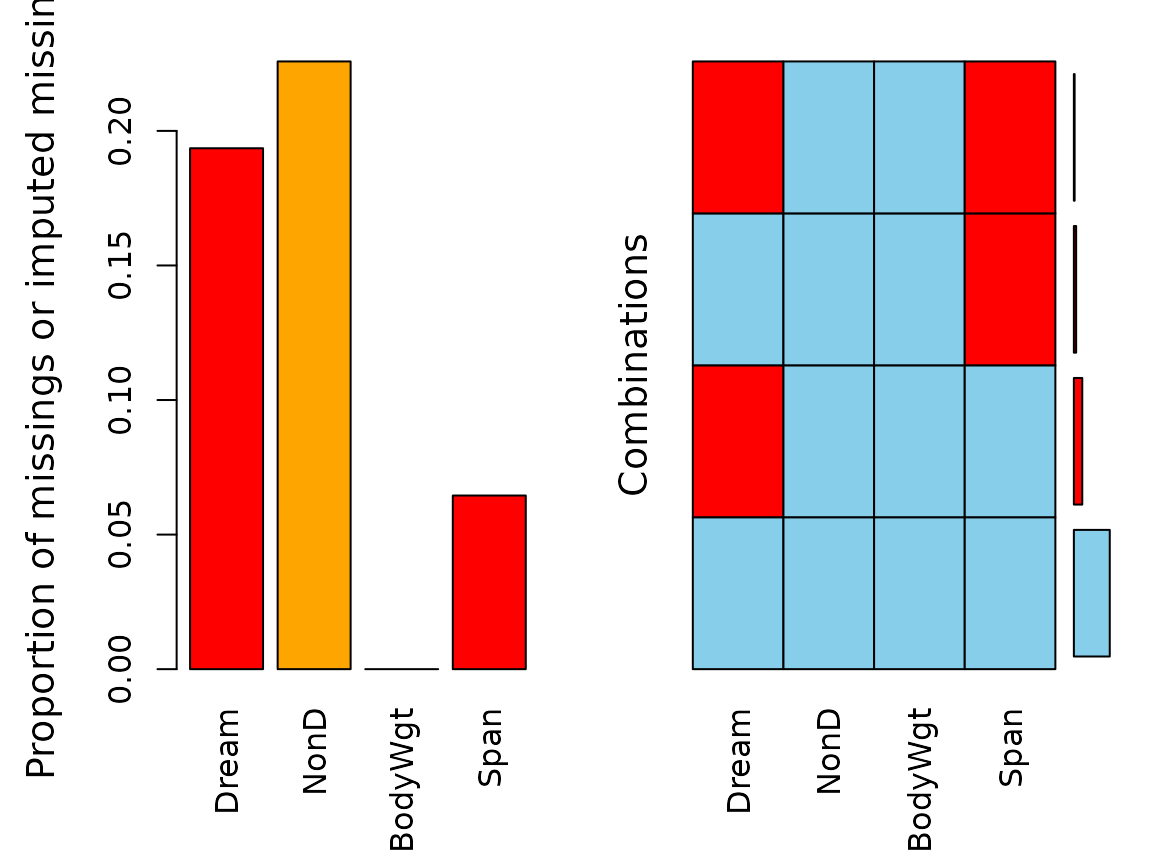We can see that kNN() imputed all missing values for NonD in our dataset. The same is true for the values imputed via hotdeck(). The specified variables in matchImpute() serve as a donor and enable imputation for NonD.

### Diagnosing the results

As we can see in the next two plots, the origninal data structure of NonD and Span is preserved by hotdeck(). kNN() reveals the typically procedure of methods, which are based on similar data points weighted by the distance.

imp_hotdeck[, c("NonD", "Span", "NonD_imp")] |>
marginplot(delimiter = "_imp")imp_knn[, c("NonD", "Span", "NonD_imp")] |>
marginplot(delimiter = "_imp")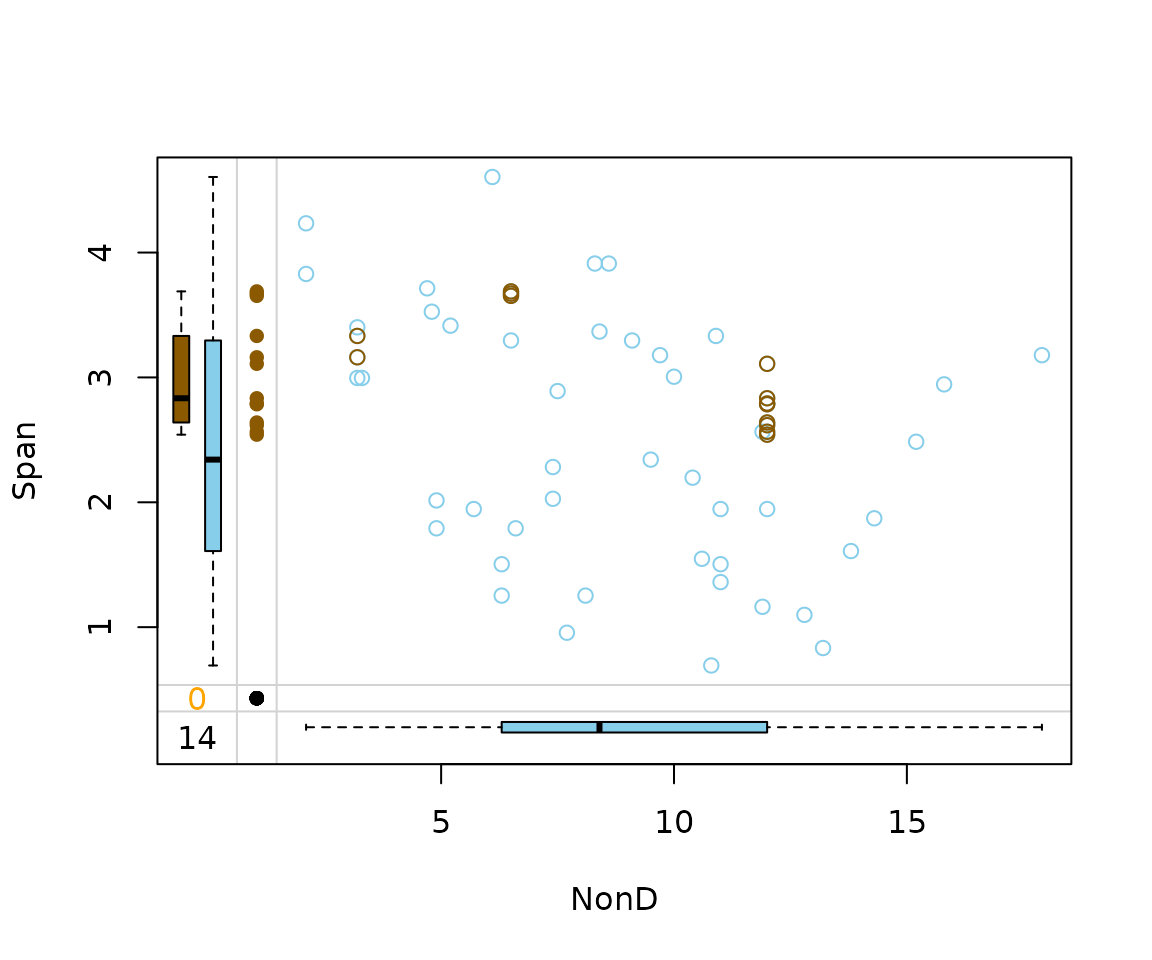matchImpute() works by sampling values from the suitable donors and also provides reasonable results.

imp_match[, c("NonD", "Span", "NonD_imp")] |>
marginplot(delimiter = "_imp")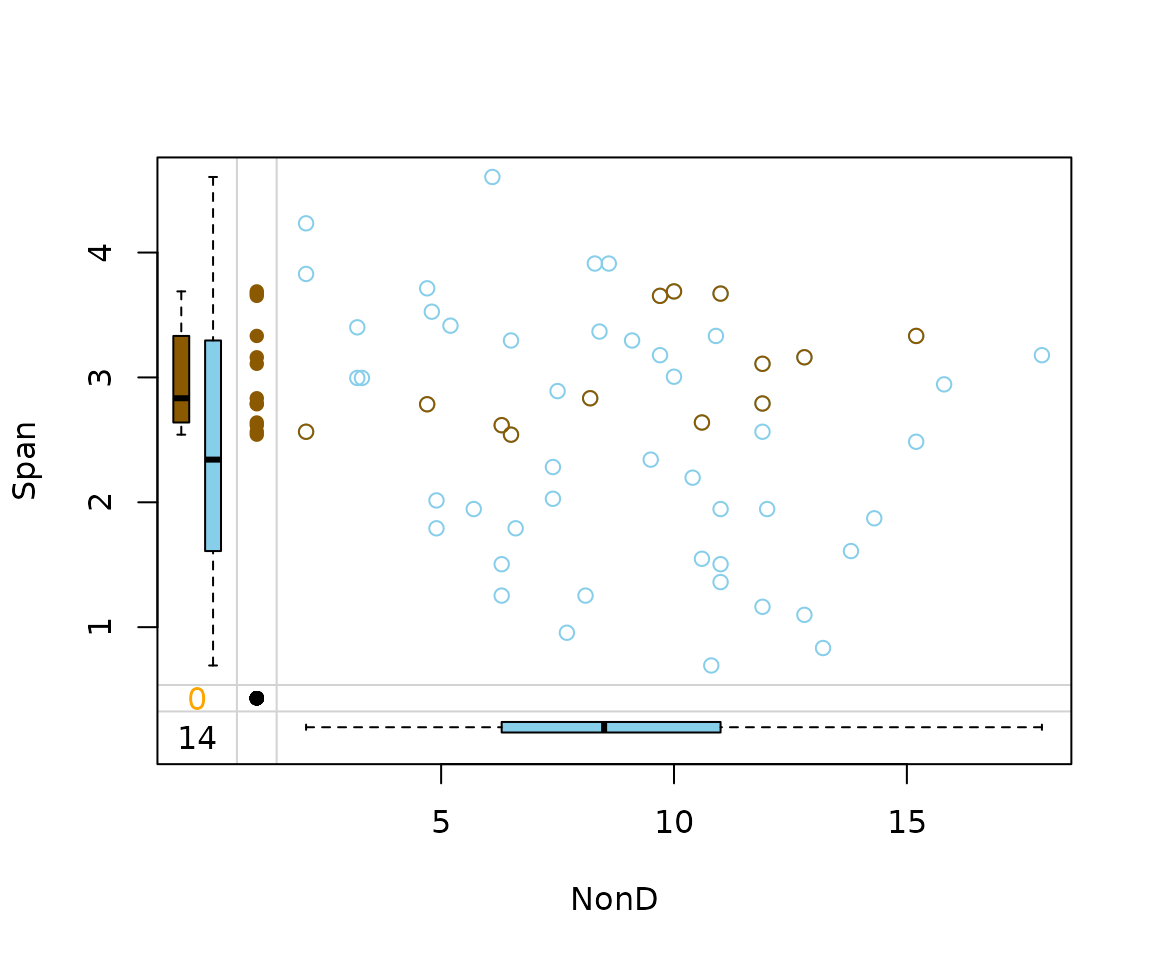## Performance of method

In order to validate the performance of kNN() and to highlight the ability to impute different datatypes the iris dataset is used. Firstly, some values are randomly set to NA.

data(iris)
df <- iris
colnames(df) <- c("S.Length","S.Width","P.Length","P.Width","Species")
# randomly produce some missing values in the data
set.seed(1)
nbr_missing <- 50
y <- data.frame(row = sample(nrow(iris), size = nbr_missing, replace = TRUE),
col = sample(ncol(iris), size = nbr_missing, replace = TRUE))
y<-y[!duplicated(y), ]
df[as.matrix(y)] <- NA

aggr(df)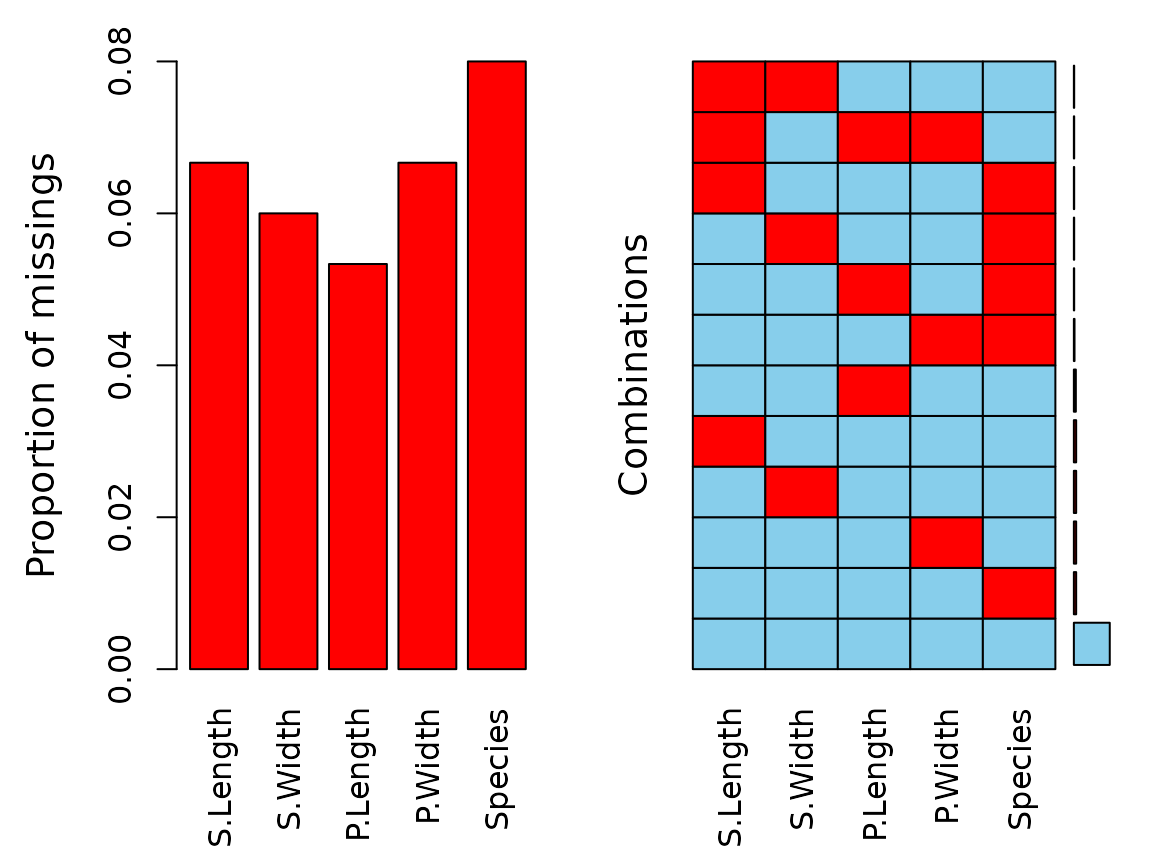sapply(df, function(x) sum(is.na(x)))
#> S.Length  S.Width P.Length  P.Width  Species
#>       10        9        8       10       12

We can see that there are missings in all variables and some observations reveal missing values on several points.

imp_knn <- kNN(df)
#>  S.Width P.Length  P.Width  S.Width P.Length  P.Width
#>      2.0      1.0      0.1      4.4      6.9      2.5
#> S.Length P.Length  P.Width S.Length P.Length  P.Width
#>      4.3      1.0      0.1      7.9      6.9      2.5
#> S.Length  S.Width  P.Width S.Length  S.Width  P.Width
#>      4.3      2.0      0.1      7.9      4.4      2.5
#> S.Length  S.Width P.Length S.Length  S.Width P.Length
#>      4.3      2.0      1.0      7.9      4.4      6.9
#> S.Length  S.Width P.Length  P.Width S.Length  S.Width P.Length  P.Width
#>      4.3      2.0      1.0      0.1      7.9      4.4      6.9      2.5
aggr(imp_knn, delimiter = "imp")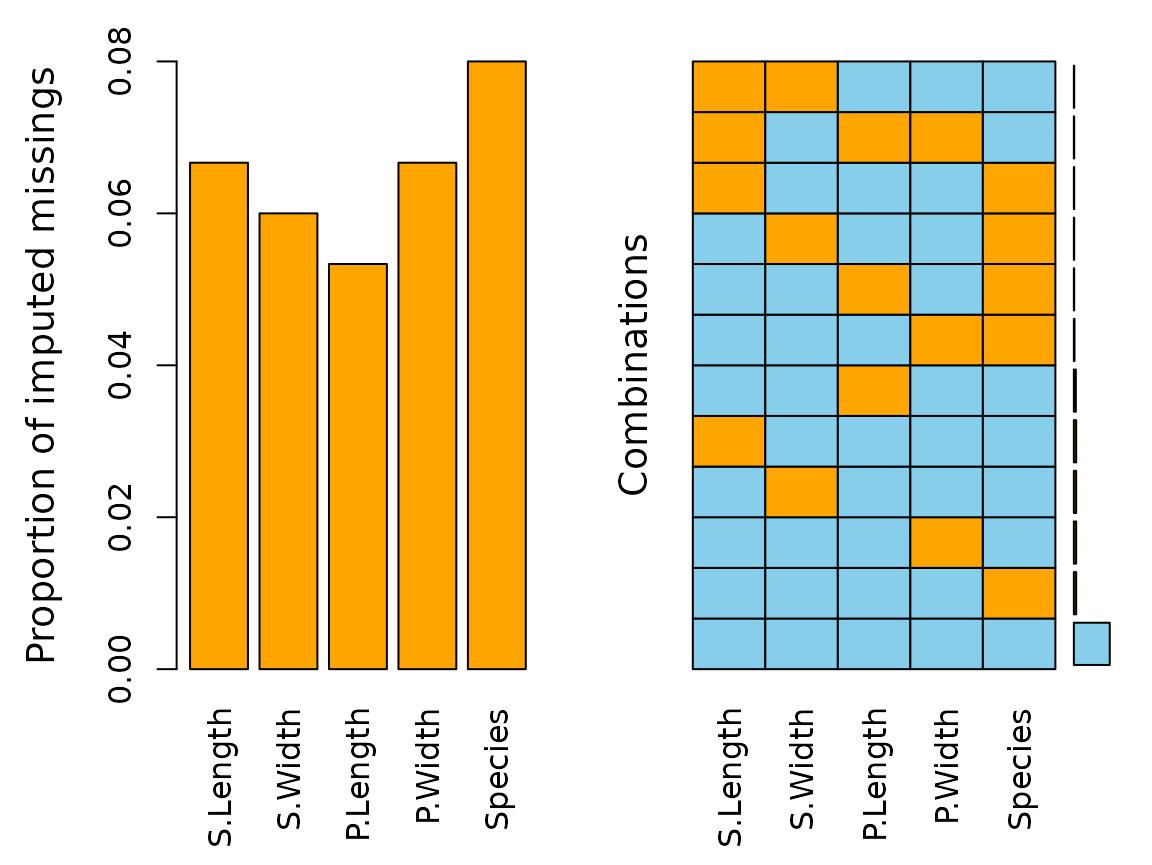The plot indicates that all missing values have been imputed by kNN(). The following table displays the rounded first five results of the imputation for all variables.